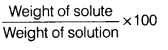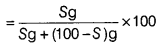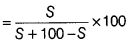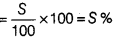If solubility of a solute is S, find its percentage by weight

If solubility of a solute is S, find its percentage by weight.

Since, solubility of a solute is given S. It means that S g solute is present in 100 g solution.
For solute, W1 = S g
For solvent, W2 = (100 - S) g
% of solute by weight in solution =